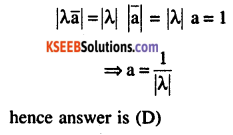# 2nd PUC Maths Question Bank Chapter 10 Vector Algebra Ex 10.3

Students can Download Maths Chapter 10 Vector Algebra Ex 10.3 Questions and Answers, Notes Pdf, 2nd PUC Maths Question Bank with Answers helps you to revise the complete Karnataka State Board Syllabus and score more marks in your examinations.

## Karnataka 2nd PUC Maths Question Bank Chapter 10 Vector Algebra Ex 10.3

### 2nd PUC Maths Vector Algebra NCERT Text Book Questions and Answers Ex 10.3

Question 1.
Find the angle between two vectors $$\overrightarrow{\mathbf{a}} \text { and } \overrightarrow{\mathbf{b}}$$ with magnitudes $$\sqrt{3}$$ and 2 respectively having $$\overrightarrow{\mathrm{a}} \cdot \overrightarrow{\mathrm{b}}=\sqrt{6}$$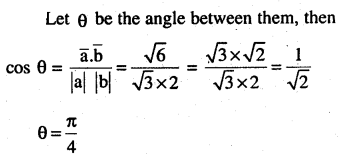Question 2.
Find the angle between the vectors
$$\hat{\mathbf{i}}-2 \hat{\mathbf{j}}+3 \hat{\mathbf{k}} \text { and } 3 \hat{\mathbf{i}}-2 \hat{\mathbf{j}}+\hat{\mathbf{k}}$$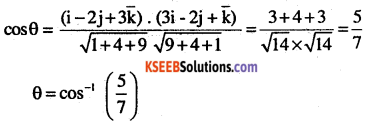Question 3.
Find the projection of the vector $$\hat{\mathbf{i}}-\hat{\mathbf{j}}$$ on the vector $$\hat{\mathbf{i}}+\hat{\mathbf{j}}$$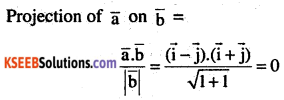Question 4.
Find the projection of the vector $$\hat{\mathbf{i}}+3 \hat{\mathbf{j}}+7 \hat{\mathbf{k}}$$ on the vector $$7 \hat{\mathbf{i}}-\hat{\mathbf{j}}+8 \hat{\mathbf{k}}$$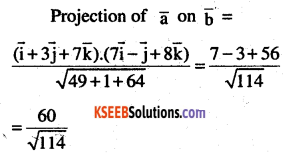Question 5.
Show that each of the given three vectors is a unit vector.
$$\frac{1}{7}(2 \hat{i}+3 \hat{j}+6 \hat{k}), \frac{1}{7}(3 \hat{i}-6 \hat{j}+2 \hat{k}), \frac{1}{7}(6 \hat{i}+2 \hat{j}-3 \hat{k})$$
Also, show that they are mutually perpendicular to each other.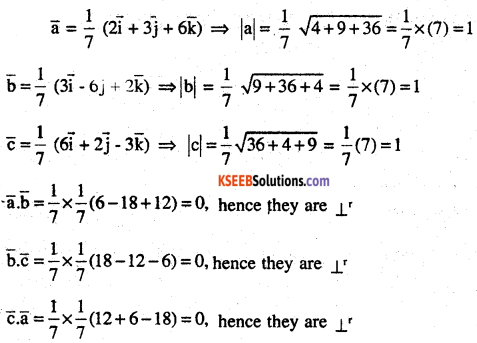Question 6.
$$\text { Find }|\overrightarrow{\mathrm{a}}| \text { and }|\overrightarrow{\mathrm{b}}|, \text { if }(\overrightarrow{\mathrm{a}}+\overrightarrow{\mathrm{b}}) \cdot(\overrightarrow{\mathrm{a}}-\overrightarrow{\mathrm{b}})=8 \text { and }|\overrightarrow{\mathrm{a}}|=8|\overrightarrow{\mathrm{b}}|$$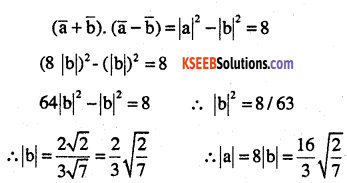Question 7.
Evaluate the product
$$(3 \vec{a}-5 \vec{b}) \cdot(2 \vec{a}+7 \vec{b})$$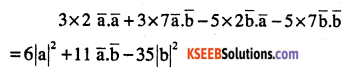Question 8.
Find the magnitude of two vectors $$\overrightarrow{\mathbf{a}} \text { and } \overrightarrow{\mathbf{b}}$$, having the same magnitude and such that the angle between them is 60° and their scalar product is 1/2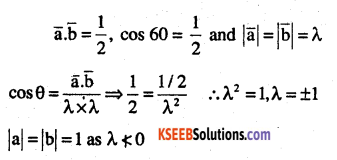Question 9.
Find $$|\overrightarrow{\mathbf{x}}|$$ if for a unit vector $$\overrightarrow{\mathrm{a}},(\overrightarrow{\mathrm{x}}-\overrightarrow{\mathrm{a}})\cdot(\overrightarrow{\mathrm{x}}+\overrightarrow{\mathrm{a}})=12$$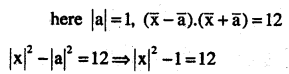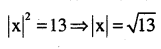Question 10.
If $$\overrightarrow{\mathrm{a}}=2 \hat{\mathrm{i}}+2 \hat{\mathrm{j}}+3 \hat{\mathrm{k}}, \overrightarrow{\mathrm{b}}=-\hat{\mathrm{i}}+2 \hat{\mathrm{j}}+\hat{\mathrm{k}}$$ and $$\overrightarrow{\mathrm{c}}=3 \hat{\mathrm{i}}+\hat{\mathrm{j}}$$ are such that $$\overrightarrow{\mathbf{a}}+\lambda \overrightarrow{\mathbf{b}}$$ is perpendicular to $$\overrightarrow{\mathbf{c}}$$, then the value of λ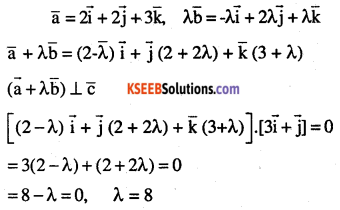Question 11.
Show that $$|\overrightarrow{\mathbf{a}}| \overrightarrow{\mathbf{b}}+|\overrightarrow{\mathbf{b}}| \overrightarrow{\mathbf{a}}$$ is a perpendicular to $$|\overrightarrow{\mathrm{a}}| \overrightarrow{\mathbf{b}}-|\overrightarrow{\mathrm{b}}| \overrightarrow{\mathrm{a}}$$, for ant two non zero vectors $$\overrightarrow{\mathbf{a}} \text { and } \overrightarrow{\mathbf{b}}$$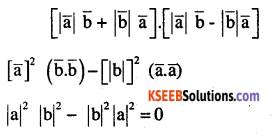Question 12.
If $$\overrightarrow{\mathrm{a}} \cdot \overrightarrow{\mathrm{a}}=0 \text { and } \overrightarrow{\mathrm{a}} \cdot \overrightarrow{\mathrm{b}}=0$$, then what can be concluded about the vector $$\overrightarrow{\mathbf{b}}$$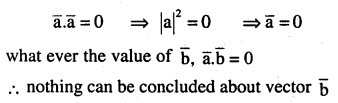Question 13.
If $$\overrightarrow{\mathrm{a}}, \overrightarrow{\mathrm{b}}, \overrightarrow{\mathrm{c}}$$are unit vector such that $$\overrightarrow{\mathbf{a}}+\overrightarrow{\mathbf{b}}+\overrightarrow{\mathbf{c}}=\overrightarrow{\mathbf{0}}$$, find the value of $$\overrightarrow{\mathrm{a}} \cdot \overrightarrow{\mathrm{b}}+\overrightarrow{\mathrm{b}} \cdot \overrightarrow{\mathrm{c}}+\overrightarrow{\mathrm{c}} \cdot \overrightarrow{\mathrm{a}}$$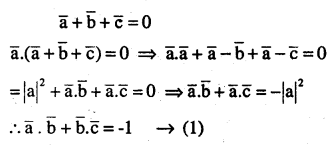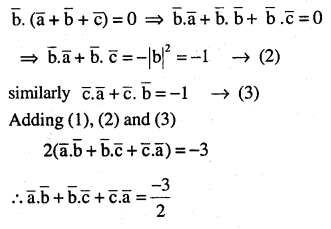Question 14.
If either vector $$\overrightarrow{\mathbf{a}}=\overrightarrow{\mathbf{0}} \quad \text { or } \quad \overrightarrow{\mathbf{b}}=\overrightarrow{\mathbf{0}}$$ then $$\overrightarrow{\mathbf{a}} \cdot \overrightarrow{\mathbf{b}}=\overrightarrow{\mathbf{0}}$$. But the converse need not be true. Justify your answer with an example.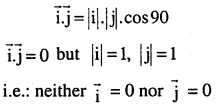Question 15.
If the vertices A, B, C of a triangle ABC are (1, 2, 3), (-1, 0, 0), (0, 1, 2), respectively, then find ∠ABC, [∠ABC is the angle between the vectors $$\bar { BA }$$ and $$\bar { BC }$$ .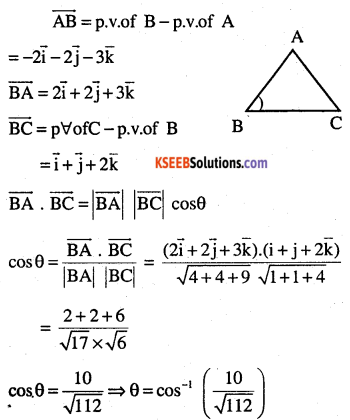Question 16.
Show that the points A (1, 2, 7), B (2, 6, 3) and C (3, 10, -1) are collinear.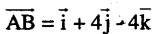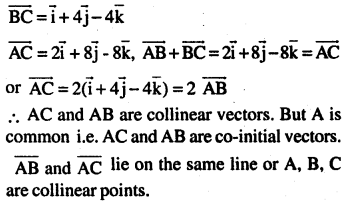Question 17.
Show that the vector$$2 \hat{i}-\hat{j}+\hat{k}, \hat{i}-3 \hat{j}-5 \hat{k}$$ and
$$\text { and } 3 \hat{i}-4 \hat{j}-4 \hat{k}$$ from the vertices of a right angled triangle: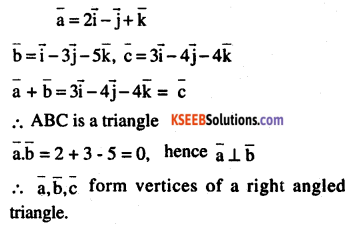If $$\overrightarrow{\mathbf{a}}$$ is a nonzero vector of magnitude ‘a’ and
λ a nonzero scalar then λ $$\vec{a}$$ is unit vector if……….